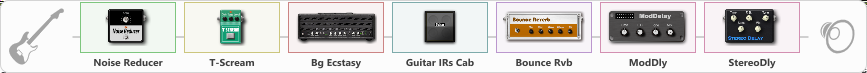Discussion in 'ToneLib-GFX presets' started by edwincrain, Mar 5, 2020.

Related video:

Effects chain:Effect: "Noise Reducer" (Dynamics / Filter), active - "yes"
{
"Sens" = 43
"Mode" = Soft
}

Effect: "T-Scream" (Overdrive / Distortion), active - "yes"
{
"Drive" = 0
"Tone" = 72
"Level" = 68
}

Effect: "Bg Ecstasy" (Amp simulators), active - "yes"
{
"Gain" = 100
"Bass" = 75
"Middle" = 82
"Treble" = 66
"Presence" = 49
"Master" = 100
"Level (dB)" = 2
}

Effect: "Guitar IRs Cab" (Cabinets), active - "yes"
{
"Model" = Mesa Rectifier (4x12")
"Mic Position" = Center
"Mic Distance" = Near
"Low Cut (Hz)" = 100
"Hi Cut (kHz)" = 10.0
"Mix" = 100
"Level (dB)" = 1
}

Effect: "Bounce Rvb" (Reverb), active - "yes"
{
"Time" = 5.0
"PreDelay" = 0
"LoDamp" = 16
"HiDamp" = 48
"Mix" = 42
}

Effect: "ModDly" (Delay), active - "yes"
{
"Time" = 409
"Feedback" = 39
"Tone" = 70
"Speed" = 2.4
"Mix" = 62
}

Effect: "StereoDly" (Delay), active - "yes"
{
"Time" = 20
"Feedback" = 7
"Tone" = 76
"Sens" = 24
"Mix" = 28
}

Note: You will need to download and install the ToneLib-GFX software to use the preset.